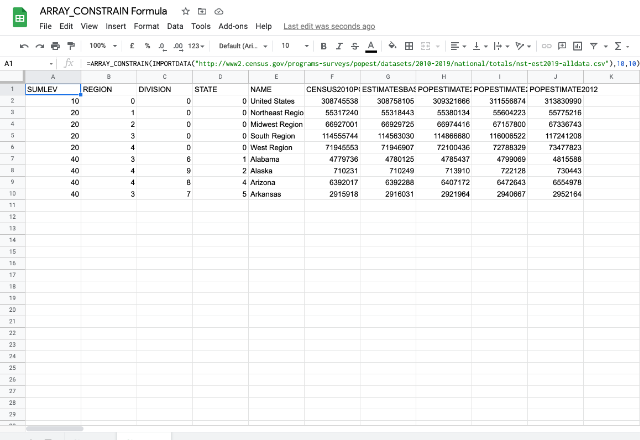# How to use the ARRAY_CONSTRAIN formula in Google SheetsIn Google Sheets, the ARRAY_CONSTRAIN formula returns a subset of the input range, characterized by the specified number of rows and columns. In other words, the formula restricts or constraints the size of input range by the specified number of rows and columns.

### Syntax

ARRAY_CONSTRAIN(input_range, num_rows, num_cols)

• input_range – can either be an address reference to the range of cells that we need to constrain, or an output range derived from another formula.
• num_rows -is the number of rows the constrained should contain.
• num_cols – is the number of columns the constrained output should contain

### Usage: ARRAY_CONSTRAIN Formula

Examples always help us digest the concepts better. Following is a sample data set on which we will try the ARRAY_CONSTRAIN formula.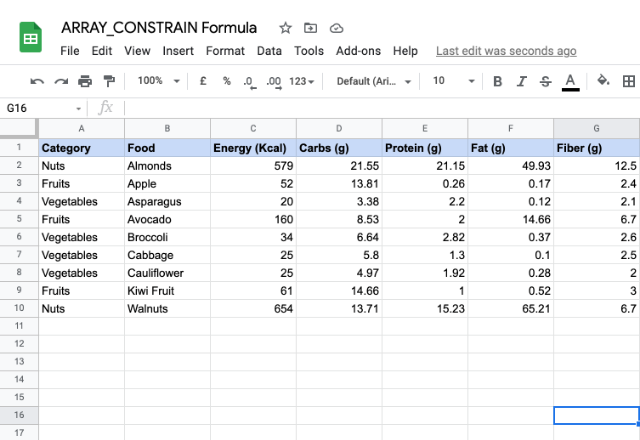Now, we keyed in the formula in cell H1, as shown in the snapshot below.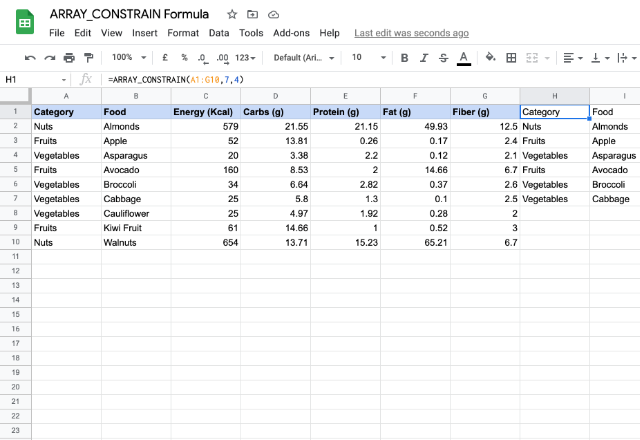The input_range is A1:G10, while the size defining parameters num_rows and num_columns are 7 and 4 respectively. We see that the output consists of 7 rows and 4 columns, exactly as we defined. It ignored the data falling beyond row 7 and column 4.

The output data flows from H1 towards right and further down. So, it is very important that we keep the cells, where we expect the data to flow, clear of any values. Otherwise, the formula returns #REF! error, as demonstrated in the screenshot below.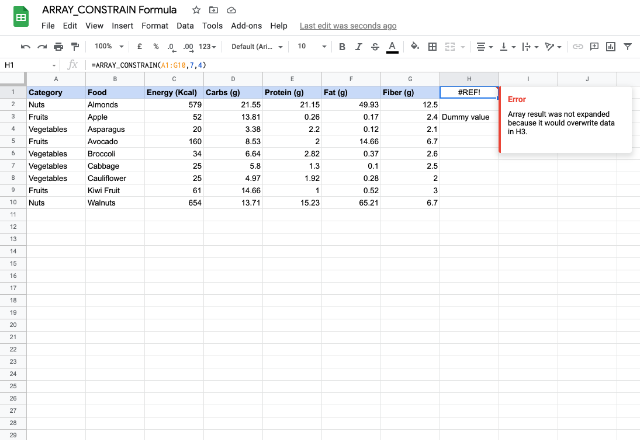Suppose that we need only the Vegetables’ data corresponding to Energy column only. Here’s how we can get creative with the formula.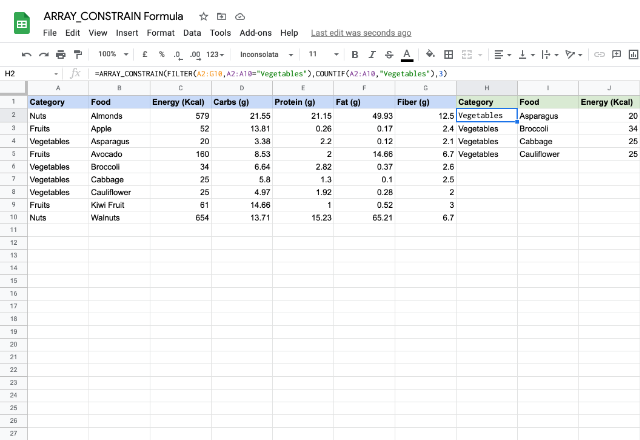Unlike the previous example, we have entered the formula in H2 here. We considered only the data rows and not the header. The FILTER formula evaluated the range for the first parameter, whereas the COUNTIF formula determined the value for the second parameter.

To take this even further we can try this formula on the ‘data import’ related functions as well. For example, here is how a normal IMPORTDATA would have looked like.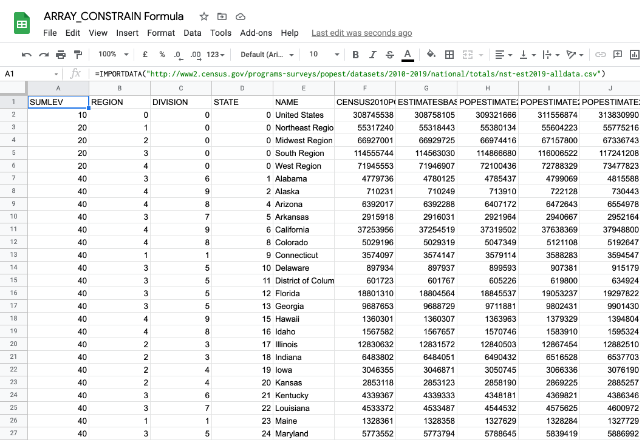In the snapshot below, we embedded this within the ARRAY_CONSTRAIN formula. And, we constrained the data to 10 rows and 10 columns.